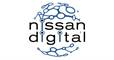Bit Flipping Game <Nissan>
Tag(s):

## Basic Programming, Basics of bit manipulation, Bit Manipulation, Bit manipulation, Easy, Implementation

Problem
Editorial
Analytics

Two players $A$ and $B$ are playing a game.They are given $N$ binary numbers as input. Each binary number is represented as a string of characters '0' or '1'. The string always ends at '1'.  In one move each player decides a bit position $p$ . Then he visits all the numbers and if their bit at that position is '1' then he changes it to '0'. It is mandatory to flip(change '1' to '0') bit of atleast one number in each move. The player who is unable to make a move loses. Player $A$ begins the game.

Input
First line contains a number $N$ as input. Next $N$ lines contain a binary string each.

Output
Print A if player A wins , B otherwise. In the next line print the move number of the last move of the winning player.

Constraints
$1 \le N \le 10^5$
$1 \le |S| \le 10^6$ where $|S|$ is sum of length of all bit strings.

Some important information -
Suppose that the length of the string $S$ in input is $K$ then $S$ is the $0^{th}$ bit , $S$ is the first bit and so on $S[k - 1]$ is the $(k - 1)^{th}$ bit. All the other bit for that string are assumed as zeros.

Note: Go through the sample explanation carefully

SAMPLE INPUT
2
01
001

SAMPLE OUTPUT
B
2

Explanation

First string in the input is 01 so its 0th bit is 0 and 1st bit is 1. Rest all the bits are zero

Second string in the input is 001 , its 0th bit is 0 , 1st bit is also 0 and second bit is 1. Rest all the bits are zero.

First move : Player A selects the bit position 1. So first string becomes 00 and second string is 001

Second move: Player B selects the bit position 2. So first string remains 00 and second becomes 000.

Now the turn is of player A but there are no numbers which have '1' in it's binary representation so A loses and B wins. Also the last move played by the player B is 2. Hence the move number is 2.

Time Limit: 1.0 sec(s) for each input file.
Memory Limit: 256 MB
Source Limit: 1024 KB
Marking Scheme: Marks are awarded when all the testcases pass.
Allowed Languages: Bash, C, C++, C++14, Clojure, C#, D, Erlang, F#, Go, Groovy, Haskell, Java, Java 8, JavaScript(Rhino), JavaScript(Node.js), Julia, Kotlin, Lisp, Lisp (SBCL), Lua, Objective-C, OCaml, Octave, Pascal, Perl, PHP, Python, Python 3, R(RScript), Racket, Ruby, Rust, Scala, Swift, Swift-4.1, TypeScript, Visual Basic

## CODE EDITOR

Initializing Code Editor...

## This Problem was Asked inChallenge Name

Nissan Digital Software Developer Hiring Challenge

OTHER PROBLEMS OF THIS CHALLENGE
• Algorithms > Searching
• Data Structures > Stacks

?